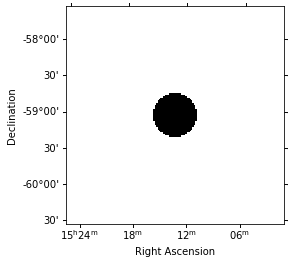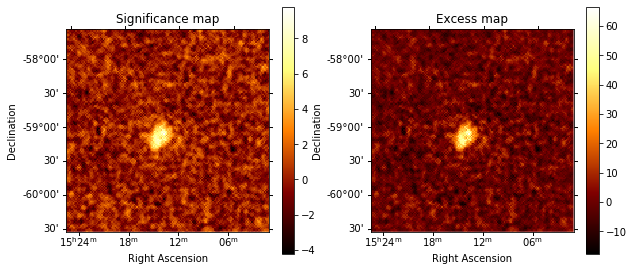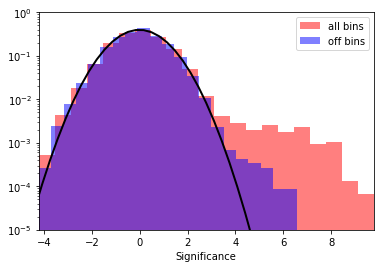This is a fixed-text formatted version of a Jupyter notebook

# Ring Background Estimation¶

## Context:¶

One of the challenges of IACT analysis is accounting for the large residual hadronic emission. An excess map, assumed to be a map of only gamma-ray events, requires a good estimate of the background. However, in the absence of a solid template bkg model it is not possible to obtain reliable background model a priori. It was often found necessary in classical cherenkov astronomy to perform a local renormalization of the existing templates, usually with a ring kernel. This assumes that most of the events are background and requires to have an exclusion mask to remove regions with bright signal from the estimation. To read more about this method, see here.

## Objective:¶

Create an excess (gamma-ray events) map of MSH 15-52 as well as a significance map to determine how solid the signal is.

## Proposed approach:¶

The analysis workflow is roughly - Compute the sky maps keeping each observation separately using the Analysis class - Estimate the background using the RingBackgroundMaker - Compute the correlated excess and significance maps using the CorrelatedExcessMapEstimator

The normalised background thus obtained can be used for general modelling and fitting.

## Setup¶

:

%matplotlib inline
import astropy.units as u
from astropy.coordinates import SkyCoord
import matplotlib.pyplot as plt
import numpy as np
from regions import CircleSkyRegion
from scipy.stats import norm

import logging

log = logging.getLogger(__name__)


Now let’s import gammapy specific classes and functions

:

from gammapy.analysis import Analysis, AnalysisConfig
from gammapy.makers import RingBackgroundMaker
from gammapy.estimators import ExcessMapEstimator
from gammapy.maps import Map
from gammapy.datasets import MapDatasetOnOff


## Creating the config file¶

Now, we create a config file for out analysis. You may load this from disc if you have a pre-defined config file.

In this example, we will use a few HESS runs on the pulsar wind nebula, MSH 1552

:

# source_pos = SkyCoord.from_name("MSH 15-52")
source_pos = SkyCoord(228.32, -59.08, unit="deg")

:

config = AnalysisConfig()
# Select observations - 2.5 degrees from the source position
config.observations.datastore = "$GAMMAPY_DATA/hess-dl3-dr1/" config.observations.obs_cone = { "frame": "icrs", "lon": source_pos.ra, "lat": source_pos.dec, "radius": 2.5 * u.deg, }  :  config.datasets.type = "3d" config.datasets.geom.wcs.skydir = { "lon": source_pos.ra, "lat": source_pos.dec, "frame": "icrs", } # The WCS geometry - centered on MSH 15-52 config.datasets.geom.wcs.fov = {"width": "3 deg", "height": "3 deg"} config.datasets.geom.wcs.binsize = "0.02 deg" # The FoV radius to use for cutouts config.datasets.geom.selection.offset_max = 3.5 * u.deg # We now fix the energy axis for the counts map - (the reconstructed energy binning) config.datasets.geom.axes.energy.min = "0.5 TeV" config.datasets.geom.axes.energy.max = "5 TeV" config.datasets.geom.axes.energy.nbins = 10 # We need to extract the ring for each observation separately, hence, no stacking at this stage config.datasets.stack = False  :  print(config)  AnalysisConfig general: log: {level: info, filename: null, filemode: null, format: null, datefmt: null} outdir: . observations: datastore:$GAMMAPY_DATA/hess-dl3-dr1
obs_ids: []
obs_file: null
obs_cone: {frame: icrs, lon: 228.32 deg, lat: -59.08 deg, radius: 2.5 deg}
obs_time: {start: null, stop: null}
datasets:
type: 3d
stack: false
geom:
wcs:
skydir: {frame: icrs, lon: 228.32 deg, lat: -59.08 deg}
binsize: 0.02 deg
fov: {width: 3.0 deg, height: 3.0 deg}
binsize_irf: 0.2 deg
selection: {offset_max: 3.5 deg}
axes:
energy: {min: 0.5 TeV, max: 5.0 TeV, nbins: 10}
energy_true: {min: 0.1 TeV, max: 10.0 TeV, nbins: 30}
map_selection: [counts, exposure, background, psf, edisp]
background:
method: null
exclusion: null
parameters: {}
methods: [aeff-default]
parameters: {}
on_region: {frame: null, lon: null, lat: null, radius: null}
containment_correction: true
fit:
fit_range: {min: 0.1 TeV, max: 10.0 TeV}
flux_points:
energy: {min: 0.1 TeV, max: 10.0 TeV, nbins: 30}
source: source
parameters: {}



## Getting the reduced dataset¶

We now use the config file to do the initial data reduction which will then be used for a ring extraction

:

%%time
# create the config
analysis = Analysis(config)

# for this specific case,w e do not need fine bins in true energy
analysis.config.datasets.geom.axes.energy_true = (
analysis.config.datasets.geom.axes.energy
)

# First get the required observations
analysis.get_observations()

Setting logging config: {'level': 'INFO', 'filename': None, 'filemode': None, 'format': None, 'datefmt': None}
Fetching observations.
Number of selected observations: 20

CPU times: user 270 ms, sys: 6.1 ms, total: 276 ms
Wall time: 278 ms

:

print(analysis.config)

AnalysisConfig

general:
log: {level: INFO, filename: null, filemode: null, format: null, datefmt: null}
outdir: .
observations:
datastore: \$GAMMAPY_DATA/hess-dl3-dr1
obs_ids: []
obs_file: null
obs_cone: {frame: icrs, lon: 228.32 deg, lat: -59.08 deg, radius: 2.5 deg}
obs_time: {start: null, stop: null}
datasets:
type: 3d
stack: false
geom:
wcs:
skydir: {frame: icrs, lon: 228.32 deg, lat: -59.08 deg}
binsize: 0.02 deg
fov: {width: 3.0 deg, height: 3.0 deg}
binsize_irf: 0.2 deg
selection: {offset_max: 3.5 deg}
axes:
energy: {min: 0.5 TeV, max: 5.0 TeV, nbins: 10}
energy_true: {min: 0.5 TeV, max: 5.0 TeV, nbins: 10}
map_selection: [counts, exposure, background, psf, edisp]
background:
method: null
exclusion: null
parameters: {}
methods: [aeff-default]
parameters: {}
on_region: {frame: null, lon: null, lat: null, radius: null}
containment_correction: true
fit:
fit_range: {min: 0.1 TeV, max: 10.0 TeV}
flux_points:
energy: {min: 0.1 TeV, max: 10.0 TeV, nbins: 30}
source: source
parameters: {}


:

%%time
# Data extraction
analysis.get_datasets()

Creating geometry.
Creating datasets.
No background maker set for 3d analysis. Check configuration.
Processing observation 20365
Processing observation 20366
Processing observation 20367
Processing observation 20368
Processing observation 20136
Processing observation 20137
Processing observation 20151
Processing observation 20282
Processing observation 20283
Processing observation 20301
Processing observation 20302
Processing observation 20303
Processing observation 20322
Processing observation 20323
Processing observation 20324
Processing observation 20325
Processing observation 20343
Processing observation 20344
Processing observation 20345
Processing observation 20346

CPU times: user 13.8 s, sys: 1.62 s, total: 15.4 s
Wall time: 15.7 s


## Extracting the ring background¶

Since the ring background is extracted from real off events, we need to use the wstat statistics in this case. For this, we will use the MapDatasetOnOFF and the RingBackgroundMaker classes.

First, we need to create an exclusion mask on the known sources. In this case, we need to mask only MSH 15-52 but this depends on the sources present in our field of view.

:

# get the geom that we use
geom = analysis.datasets.counts.geom
energy_axis = analysis.datasets.counts.geom.get_axis_by_name("energy")
geom_image = geom.to_image().to_cube([energy_axis.squash()])

regions = CircleSkyRegion(center=source_pos, radius=0.3 * u.deg)For the present analysis, we use a ring with an inner radius of 0.5 deg and width of 0.3 deg.

:

ring_maker = RingBackgroundMaker(
)


### Create a stacked dataset¶

Now, we extract the background for each dataset and then stack the maps together to create a single stacked map for further analysis

:

#%%time
stacked_on_off = MapDatasetOnOff.create(geom=geom_image)
for dataset in analysis.datasets:
dataset_image = (
dataset.to_image()
)  # Ring extracting makes sense only for 2D analysis
dataset_on_off = ring_maker.run(dataset_image)
stacked_on_off.stack(dataset_on_off)

/Users/terrier/Code/anaconda3/envs/gammapy-dev/lib/python3.7/site-packages/astropy/units/quantity.py:481: RuntimeWarning: invalid value encountered in true_divide
result = super().__array_ufunc__(function, method, *arrays, **kwargs)


This stacked_on_off has on and off counts and acceptance maps which we will use in all further analysis. The acceptance and acceptance_off maps are the system acceptance of gamma-ray like events in the on and off regions respectively.

:

print(stacked_on_off)

MapDatasetOnOff
---------------

Name                            : U95u2STT

Total counts                    : 40051
Total predicted counts          : nan
Total background counts         : nan

Exposure min                    : 2.34e+09 m2 s
Exposure max                    : 8.18e+09 m2 s

Number of total bins            : 22500
Number of fit bins              : 22500

Fit statistic type              : wstat
Fit statistic value (-2 log(L)) : nan

Number of models                : 0
Number of parameters            : 0
Number of free parameters       : 0

Total counts_off                : 88113384
Acceptance                      : 22500
Acceptance off                  : 49447599



## Compute correlated significance and correlated excess maps¶

We need to convolve our maps with an apprpriate smoothing kernel. The significance is computed according to the Li & Ma expression for ON and OFF Poisson measurements, see here. Since astropy convolution kernels only accept integers, we first convert our required size in degrees to int depending on our pixel size.

:

# Using a convolution radius of 0.04 degrees
estimator = ExcessMapEstimator(0.04 * u.deg)
lima_maps = estimator.run(stacked_on_off, steps="ts")

:

significance_map = lima_maps["significance"]
excess_map = lima_maps["excess"]

:

# We can plot the excess and significance maps
plt.figure(figsize=(10, 10))
ax1 = plt.subplot(221, projection=significance_map.geom.wcs)
ax2 = plt.subplot(222, projection=excess_map.geom.wcs)

ax1.set_title("Significance map")

ax2.set_title("Excess map")

:

(<Figure size 720x720 with 4 Axes>,
<matplotlib.axes._subplots.WCSAxesSubplot at 0x126560630>,
<matplotlib.colorbar.Colorbar at 0x11b81b860>)It is often important to look at the signficance distribution outside the exclusion region to check that the background estimation is not contaminated by gamma-ray events. This can be the case when exclusion regions are not large enough. Typically, we expect the off distribution to be a standard normal distribution.

:

# create a 2D mask for the images
significance_all = significance_map.data[np.isfinite(significance_map.data)]
significance_off = significance_map_off.data[
np.isfinite(significance_map_off.data)
]

plt.hist(
significance_all,
density=True,
alpha=0.5,
color="red",
label="all bins",
bins=21,
)

plt.hist(
significance_off,
density=True,
alpha=0.5,
color="blue",
label="off bins",
bins=21,
)

# Now, fit the off distribution with a Gaussian
mu, std = norm.fit(significance_off)
x = np.linspace(-8, 8, 50)
p = norm.pdf(x, mu, std)
plt.plot(x, p, lw=2, color="black")
plt.legend()
plt.xlabel("Significance")
plt.yscale("log")
plt.ylim(1e-5, 1)
xmin, xmax = np.min(significance_all), np.max(significance_all)
plt.xlim(xmin, xmax)

print(f"Fit results: mu = {mu:.2f}, std = {std:.2f}")

Fit results: mu = -0.02, std = 1.00[ ]:


`